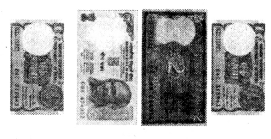# PSEB 4th Class Maths Solutions Chapter 4 Money (Currency) Ex 4.1

Punjab State Board PSEB 4th Class Maths Book Solutions Chapter 4 Money (Currency) Ex 4.1 Textbook Exercise Questions and Answers.

## PSEB Solutions for Class 4 Maths Chapter 4 Money (Currency) Ex 4.1

1. Draw 50 paise coins for given notes/coins :

Question 1.Solution: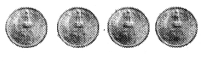= Take four coins of 50 paise

Question 2.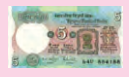Solution: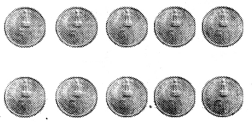= Take ten coins of 50 paiseQuestion 3.Solution: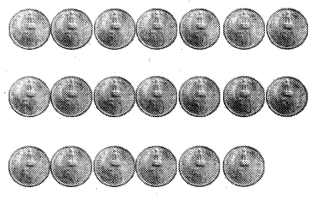= Take twenty coins of 50 paise

Question 4.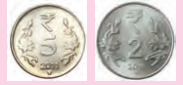Solution: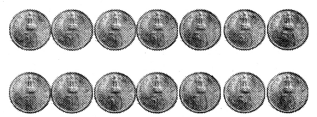= Take fourteen coins of 50 paise

Question 5.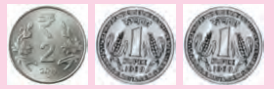Solution: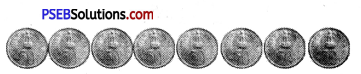= Take eight coins of 50 paise.

2. Draw notes/coins, converting 50 paise into rupees.

Question 1.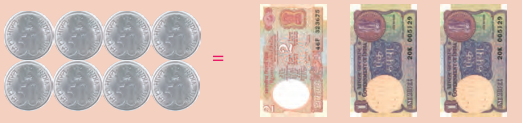Question 2.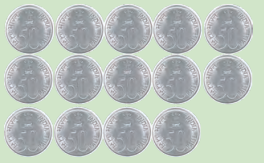Solution: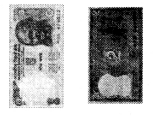Question 3.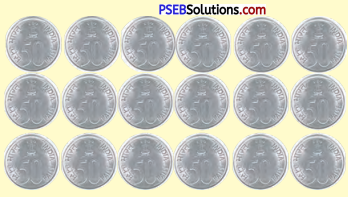Solution: